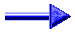## Sequences

### Introduction

At april 17, 2004, during the NMC2004, Neil Sloane presented a lecture about sequences and his online integer sequences site. After the lecture I spoke to Neil, and I promised him to send some missing sequences. These sequences can be found below. If you would like to contact me about these sequences, you can send a message to matthijsssequences. Unfortunately I have not so much time to do research. I have ideas for a lot of new projects and I found a lot of unknown sequences.

### A067957

describes the number of permutations a_1,a_2,...,a_n of 1,2,...,n such that for all j=1,2,...,n: a_j|SUM_{i=1}^{i=j}a_i.
NEW_2 : 0, 1, 1, 0, 0, 0, 0, 0, 0, 0, 0, 0, 0, 0, 0, 0, 0, 0, 0, 0, 0, 0, 0, 0, 0, 0, 0, 0, 0, 0, 0, 0, 0, 0, 0, 6, 6, 1, 11, 9, 15, 14, 14, 23, ... describes the number of the subsets of permutations starting with 2.
NEW_3 : 0, 0, 1, 1, 1, 0, 0, 0, 0, 0, 0, 0, 0, 0, 0, 0, 0, 0, 0, 0, 0, 0, 0, 0, 0, 0, 1, 0, 0, 0, 0, 0, 0, 0, 12, 20, 20, 1, 163, 55, ... describes the number of the subsets of permutations starting with 3.
NEW_4 : 0, 0, 0, 1, 2, 2, 2, 0, 0, 0, 0, 1, 1, 0, 1, 1, 1, 0, 0, 0, 0, 0, 0, 6, 8, 3, 14, 12, 18, 13, 14, 6, 26, 13, 198, 152, 220, 118, 1033, 807,... describes the number of the subsets of permutations starting with 4.

### A093387

0, 0, 1, 2, 6, 12, 29, 58, 130, 260, 562, 1124, 2380, 4760, 9949, 19898, 41226, 82452, 169766, 339532, 695860, 1391720, 2842226, 5684452, 11576916, 23153832, 47050564, 94101128, 190876696, 381753392, 773201629, 1546403258, 3128164186, 6256328372, 12642301534
2^(n-1)-binomial(n, floor(n/2),statements.zip

### A093388

1, 6, 42, 312, 2394, 18756, 149136, 1199232, 9729882, 79527084, 654089292, 5408896752, 44941609584, 375002110944, 3141107339328, 26402533581312, 222635989516122, 1882882811380284, 15967419789558804, 135752058036988848, 1156869080242393644
(n+1)^2 a_{n+1} = (17n^2+17n+6) a_n - 72n^2 a_{n-1}, this result was written down in my (master)thesis, august 26, 1983, unfortunately unpublished.

### A094062

A camel can carry one banana at a time on his back. It is on diet and therefore can only have one banana at a time in its stomach. As soon as it has eaten a banana it walks a mile and then needs a new banana (in order to be able to continue its iterary).
Let there be a stock of N bananas at the border of the desert. How far can the camel penetrate into the desert? (Of course it can form new stocks with transported bananas.)
Question: How many bananas are needed to penetrate (at least) k miles? We get the sequence : 1, 2, 6, 22, 83, ...camel.zip

Remark from Michiel de Bondt (debondt_#at#_math.kun.nl):

I think that \$a(n) := ceil((3-sqrt(3))*4^(n-2)+1)\$ is a better formula than your formula \$ceil((3-sqrt(3))*4^(n-3))+1\$. Another formula which looks better is \$b(n) := ceil((3-sqrt(3))*4^(n-2-1/16^(n-3/2))+1)\$. See Original text (in Dutch).

Another remark from Michiel de Bondt (debondt_#at#_math.kun.nl):

The problem can be reformulated in terms of a jeep and complete refills. See http://www.inf.fu-berlin.de/inst/ag-ti/publications/rote.en.html#AREA01 Optimal logistics for expeditions-the jeep problem with complete refilling.

### A098853

At october 11, 2004 I submitted a sequence about Egyptian fractions.
Let the Egyptian algorithm applied on the quotient p/q be defined by
1. substract the largest quotient 1/n smaller or equal then p/q.
2. if not zero go to (1).
Consider the smallest denominator q such that on n/q the Egyptian algorithm can be applied n times. Since the n-th term is of the form k*n+1, only k has been written down.
0, 1, 2, 4, 6, 18, 36, 12, 30, 162, 18, 330, 136, 858, 1092, 198, 1470

I would like to thank Greg Martin for his reply on this sequence.

### A new sequence

Suppose you have to solve a crossword-puzzle of n x n. You have to fill it by the digits 0,...,9 and all the descriptions are equal, namely square of a number. How would you solve this crossword-puzzle (let's say crosssquare-puzzle)? Well the 1 x 1 crosssquare-puzzle is easy. Only 1, 4, 9 are the solutions. If you are interested here are the solutions for 2, number of solutions : 4, the solutions for 3, number of solutions : 13, the solutions for 4, number of solutions : 14, the solutions for 5, number of solutions : 76, the solutions for 6, number of solutions : 40, the solutions for 7, number of solutions : 459. So therefore I introduce the sequence of number of solutions: 3, 4, 13, 14, 76, 40, 459, ...

(By the way, only the symmetric solutions are given.)

I wrote a program in Delphi (Pascal) under Windows.

While I checked the program I found that the results differ from the program and that there are no asymmetric solutions shown. I remember that it took too much time for my computer to find asymmetric solutions. But now computers are faster, and probably the program could be made faster.

My program started with the most right column, and works from right/bottom to left/top (except of the first row and column).sequences.zip